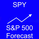This chart is an example in which price dropped lower than the Fibonacci retracement numbers. Price did not gap down to each successive Fibonacci number. Instead it sampled almost all of the prices on the way down and through the Fibonacci numbers and turned at non-fibonacci numbers. Therefore, this chart is a great example to show that stocks do not move according to Fibonacci numbers.